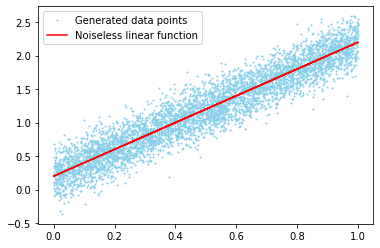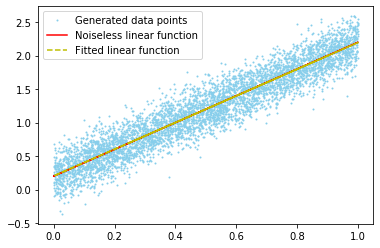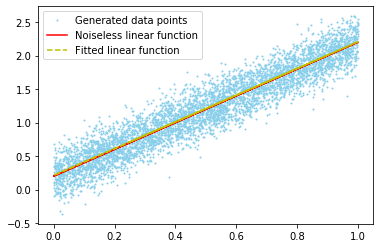# Hands-On: Linear Regression with BigDL¶

### AI and Predictive Analytics in Data-Center Environments - http://dcai.bsc.es¶

In the previous hands-on we saw how to train a Linear Regression model with Spark ML, now we will do the same with BigDL as an introduction of the BigDL workflow.

Although it's meant for deep learning and Big Data applications, BigDL can also be used for applications like a simple linear regression so we will seize that fact to illustrate the different BigDL constructs and data structures without having to deal with the complexity of deep neural networks.

## Getting a Spark Context¶

As in our setup we will be running BigDL in local mode we need to create a Spark Context before proceeding:

In :
from bigdl.util.common import *
from pyspark import SparkContext

sc = SparkContext.getOrCreate(conf=create_spark_conf().setMaster("local[*]"))
sc

Prepending /home/fjjm/.local/share/virtualenvs/exercises-oJA80Xyq/lib/python3.7/site-packages/bigdl/share/conf/spark-bigdl.conf to sys.path

Out:

SparkContext

Spark UI

Version
v2.4.4
Master
local[*]
AppName
pyspark-shell

## Generating the data¶

As in the previous example, we will generate a data sample with a obvious linear relationship:

In :
import numpy as np
import matplotlib.pyplot as plt

# Set the seed for repeatability
seed = 2019
np.random.seed(seed)

# Generate the dataset
y0 = 0.2
m = 2
noise_var = 0.2
num_samples = 5000
x = np.random.uniform(0, 1, num_samples)
y = y0 + m*x + np.random.normal(0, noise_var, num_samples)

# Plot the data to check that everything went well
plt.plot(x, y, 'bo', markersize=1, label="Generated data points", color="skyblue");
plt.plot(x, y0 + m*x, 'r-', label="Noiseless linear function");
plt.legend();## Starting the BigDL engine¶

BigDL requires certain environment variables to be set in order to run properly, so you need to initialize the BigDL engine before calling any of its methods by running init_engine():

In :
from bigdl.util.common import init_engine
init_engine()


## The BigDL Modelling Framework¶

BigDL is a deep learning library and therefore it is designed for dealing with neural networks. All models in BigDL are built as a series of layers defining a neural network, simpler models (as our linear regression) being a degenerate cases of those (eg. a single layer with a single neuron). BigDL implements a wide array of different layers that can be included in your model, like processing, transforming (preprocessing), activation functions and many other types of layer.

BigDL offers two different APIs for defining models, the Sequential API and the Functional API:

### Sequential API¶

As its name implies, the Sequential API allows to build models by defining its layers in a sequential fashion. In order to arrange the layers together and manage their connections BigDL defines a series of containers that can be populated with layers and treated as a single processing block. It also allows to connect different containers to create complex models.In order to implement a linear regression in BigDL we only need a single Linear layer, so we can go without actually defining it inside a container. Taking into account that the model has a single input and a single output, it is defined as follows:

In :
from bigdl.nn.layer import Linear
num_inputs = 1
num_outputs = 1
seq_model = Linear(num_inputs, num_outputs)

creating: createLinear


### Functional API¶

The Functional API allows to define models in a graph-like fashion by describing the connections between different layers.The BigDL team recommends the Functional API to build complex models due to its increased flexibility over the Sequential API. In order to build our linear regression model we need to create a linear layer node (Notice the additional () when declaring the linear layer) and add it to a Model object. As we only have one layer, we need to pass it both as input and output:

In :
from bigdl.nn.layer import Model
lin = Linear(num_inputs, num_outputs)()
fun_model = Model([lin], [lin])
print(fun_model.layers)

creating: createLinear
creating: createModel
Linear[6e6a8de7](1 -> 1)


### Model Parameters¶

Model parameters (weights and bias/intercept) can be accessed through the method get_weights():

In :
print(fun_model.get_weights())
# The first element corresponds to the linear regression's coefficients:
print("Coefficients: {}".format(fun_model.get_weights()))
# The second element corresponds to the linear regression's bias or intercept:
print("Intercept: {}".format(fun_model.get_weights()))

[array([[-0.8132351]], dtype=float32), array([0.9693888], dtype=float32)]
Coefficients: [[-0.8132351]]
Intercept: [0.9693888]


For Functional models, get_parameters() has to be called on the model itself rather than on the nodes containing the layers. Sequential models allows to call it both in the container and the individual layers. If a model contains more than one layer, it will return the weights as a sequence of weight, bias for each one of the layers:

## Training Alternatives¶

For model training and validation BigDL offers two alternatives: Either use BigDL's own data structures and methods ( Heavily inspired by Torch ) or integrate the model into a Spark ML pipeline thanks to ML Pipeline, a high level API that wraps BigDL models and allows to use them as Spark ML transformers.

Although both methods use the same base model there are several differences in how to preprocess the data and validate the model, so let's start by learning how to do it with just BigDL methods.

## Training a model with bare BigDL¶

### Preparing the data¶

The first step in creating our BigDL linear regression model is to transform the data into the appropriate format. BigDL uses Spark's RDD as its data structure of choice to train models and make predictions, with each element in the RDD being a BigDL Sample. Samples are sequences of Tensor (BigDL's implementation of Torch's Tensor) that represent a single record of the dataset. They are comprised of a feature and a label field, representing the record's features (as a single vector) and its corresponding label respectively. You can build a Sample from Numpy arrays containing the features and label by calling Sample.from_ndarray():

Important: The BigDL team instructs to always use Sample.from_ndarray() to construct a Sample from Numpy arrays in Python.

In :
from bigdl.util.common import Sample
from bigdl.util.common import *
from pyspark import SparkContext
from bigdl.nn.layer import *
import bigdl.version
sc = SparkContext.getOrCreate(conf=create_spark_conf().setMaster("local[*]"))
samples = [Sample.from_ndarray(np.array(feat), np.array(label)) for feat, label in zip(x,y)]
data_rdd = sc.parallelize(samples)
data_rdd.take(5)

Out:
[Sample: features: [JTensor: storage: [0.9034822], shape: , float], labels: [JTensor: storage: [2.3289778], shape: , float],
Sample: features: [JTensor: storage: [0.3930805], shape: , float], labels: [JTensor: storage: [0.87209755], shape: , float],
Sample: features: [JTensor: storage: [0.62397], shape: , float], labels: [JTensor: storage: [1.7100179], shape: , float],
Sample: features: [JTensor: storage: [0.6378774], shape: , float], labels: [JTensor: storage: [1.7522986], shape: , float],
Sample: features: [JTensor: storage: [0.88049906], shape: , float], labels: [JTensor: storage: [2.334894], shape: , float]]

Once the data is in the right format we can split it into train and test instances:

In :
train_test_rate = 0.7
train_rdd, test_rdd = data_rdd.randomSplit([train_test_rate, 1-train_test_rate], seed)
print("Train rows: {}".format(train_rdd.count()))
print("Test rows: {}".format(test_rdd.count()))

Train rows: 3475
Test rows: 1525


### Training the model¶

Models in BigDL are trained through an Optimizer, which takes at least the following parameters:

• Model: Neural network model to train. May be a layer, a sequence of layer or a graph of layers.
• Data: Training data, in RDD of Sample format.
• Loss Function: Function that compares the model output with the training labels and provides a measure of how good the models fit the data.
• Stopping Criteria: A rule for the optimizer to know when to stop training.
• Batch Size: Size of the batch of data used to train the model at each iteration. This batch is sampled with repetition from the training data. Important: The batch size must be a multiple of the number of cores available.

Optionally you can also provide an Optimization Method, which allows you to define the training rate at which you want the network to train. If not specified, it defaults to Stochastic Gradient Descent in supervised learning:

In :
from bigdl.optim.optimizer import Optimizer
from bigdl.nn.criterion import *
from bigdl.optim.optimizer import *

batch_size = 16
epochs = 10
learning_rate=0.2

optimizer = Optimizer(model = fun_model,
training_rdd = train_rdd,
criterion = MSECriterion(),
end_trigger = MaxEpoch(epochs),
optim_method=SGD(learningrate=learning_rate),
batch_size = batch_size)

creating: createMSECriterion
creating: createMaxEpoch
creating: createDefault
creating: createSGD
creating: createDistriOptimizer


You can tell the optimizer to validate the model against a given dataset (normally the test data) during the training process by setting a validation policy:

In :
optimizer.set_validation(
batch_size=batch_size,
val_rdd=test_rdd,
trigger=EveryEpoch(),
val_method=[Loss(MSECriterion())]
)

creating: createEveryEpoch
creating: createMSECriterion
creating: createLoss


In order to analize and visualize the training (and validation) process you can enable logging. As with the validator, we simply need to initialize the training and validation logs and indicate the optimizer to use them:

In :
import datetime as dt
log_dir = '/tmp/bigdl_summaries'
app_name = 'linreg-' + dt.datetime.now().strftime("%Y%m%d-%H%M%S")

# Create the train and validation summaries
train_summary = TrainSummary(log_dir=log_dir, app_name=app_name)\
.set_summary_trigger("Parameters", SeveralIteration(50))
val_summary = ValidationSummary(log_dir=log_dir, app_name=app_name)

# Pass them to the optimizer
optimizer.set_train_summary(train_summary)
optimizer.set_val_summary(val_summary)
print("Logs saved to: {}/{}".format(log_dir, app_name))

creating: createTrainSummary
creating: createSeveralIteration
creating: createValidationSummary
Logs saved to: /tmp/bigdl_summaries/linreg-20200204-163413


Once everything is set up we can finally train our model by simply calling optimize():

In :
train_summary.methods

Out:
<py4j.java_gateway.JavaMember at 0x7f8668fbc390>
In :
optimizer.optimize();


Note that unlike Spark ML's fit() method, optimize() does not return the trained model but rather updates the coefficients of the model instance it's training.

### Validating the model¶

You can evaluate the trained model against the test data using the evaluate method and passing the approapriate loss function:

In :
test_results = fun_model.evaluate(test_rdd, batch_size, [Loss(MSECriterion())])
print(test_results)

creating: createMSECriterion
creating: createLoss
Evaluated result: 0.039737116545438766, total_num: 765, method: Loss


If we pull the linear regression coefficients from the model we can see it has managed to fit quite well the parameters of the function used to generate the data (recall that we generated the data from a linear function with slope 2 and intercept 0.2):

In :
print("Coefficients: {}".format(fun_model.get_weights()))
print("Intercept: {}".format(fun_model.get_weights()))

Coefficients: [[1.9928486]]
Intercept: [0.20330335]


Plotting the fitted line against the original data, we can see that it indeed matches almost perfectly the generating function:

In :
plt.plot(x, y, 'bo', markersize=1, label="Generated data points", color="skyblue");
plt.plot(x, y0 + m*x, 'r-', label="Noiseless linear function");
plt.plot(x, fun_model.get_weights() + fun_model.get_weights()*x, 'y--', label="Fitted linear function");
plt.legend();## Training a model with ML Pipeline¶

BigDL provides a high level API to train BigDL models with the Spark ML Estimator/Transformer pattern. This allows to integrate BigDL models into the Spark ML pipeline workflow, being specially convenient if you want to include deep learning models into an existing pipeline and don't want to rewrite big chunks of it.

### Preparing the data¶

Having BigDL integrated in the BigDL pipeline also means that we can work with DataFrames rather than dealing with RDDs of Sample. Let's then create a DataFrame out of our data in the same way we did in the Spark ML Linear Regression notebook:

In :
from pyspark.sql import SparkSession

spark = SparkSession \
.builder \
.config("master", "local[*]") \
.getOrCreate()

In :
from pyspark.ml.feature import VectorAssembler
train_test_rate = 0.7
data_df = spark.createDataFrame(np.column_stack([x,y]).tolist(),['x', 'y'])
train_df, test_df = VectorAssembler(inputCols = ['x'], outputCol = 'features')\
.transform(data_df)\
.selectExpr("features", "y as label")\
.randomSplit([train_test_rate, 1-train_test_rate], seed)

print("Train rows: {}".format(train_df.count()))
print("Test rows: {}".format(test_df.count()))

Train rows: 3509
Test rows: 1491


As we already went through the DataFrame creation process in the previous notebook, we have used a more succint approach here by chaining the transformation and selection commands into a single, long instruction.

### Training the model¶

To get our Spark ML-friendly linear regression model we need to wrap up a BigDL model with a DLEstimtor. The DLEstimator object allows to call the fit() method used in Spark ML and returns a DLModel object containing the trained model on which transform() can be called. In the same way as Optimizer, DLEstimator requires several additional parameters:

In :
from bigdl.dlframes.dl_classifier import DLEstimator
# Because the Functional API model has been already trained, we'll take the secuential one
lr_ml = DLEstimator ( model = seq_model,
criterion = MSECriterion(),
feature_size = [num_inputs],
label_size = [num_outputs])\
.setBatchSize(batch_size)\
.setMaxEpoch(epochs)\
.setLearningRate(learning_rate)

creating: createMSECriterion
creating: createDLEstimator


You can see that DLEstimator does not take a batch size, stopping criterion or learning rate as a parameter, so we need to set them by calling the respective functions.

The model is now ready for training:

In :
lr_model = lr_ml.fit(train_df)


Just like in Spark ML we can now make predictions by calling transform on lr_model and passing it the appropriate data in DataFrame form:

In :
pred_train = lr_model.transform(train_df)
pred_train.show(5)

+--------------------+-------------------+--------------------+
|            features|              label|          prediction|
+--------------------+-------------------+--------------------+
|[0.00583752758798...| 0.5974935870984734|[0.22417478263378...|
|[0.00692086824895...|0.47763390326476124|[0.22633564472198...|
|[0.01504372654691...| 0.5711796582656647|[0.2425377517938614]|
|[0.01540782054721...|0.09392341924410447|[0.24326397478580...|
|[0.01547159109614...|0.21871977213766364|[0.24339118599891...|
+--------------------+-------------------+--------------------+
only showing top 5 rows



The output of transform is a DataFrame, so we can handle it as we did in the Spark ML section. Notice that each row in the prediction column contains a vector rather than a single value. Because that can cause issues further down the line, let's put the prediction column in scalar form:

In :
import pyspark.sql.functions as f
pred_train = pred_train.withColumn("prediction", f.explode(f.col('prediction')))
pred_train.show(5)

+--------------------+-------------------+-------------------+
|            features|              label|         prediction|
+--------------------+-------------------+-------------------+
|[0.00583752758798...| 0.5974935870984734|0.22417478263378143|
|[0.00692086824895...|0.47763390326476124|0.22633564472198486|
|[0.01504372654691...| 0.5711796582656647| 0.2425377517938614|
|[0.01540782054721...|0.09392341924410447|0.24326397478580475|
|[0.01547159109614...|0.21871977213766364|0.24339118599891663|
+--------------------+-------------------+-------------------+
only showing top 5 rows



The method withColumn() transform a DataFrame by adding (or replacing if you provide an existing column's name) a column containing the results of the column transformation indicated by the second parameter. The explode() method separates a vector into its elements.

We can now get some statistics the Spark ML way:

In :
from pyspark.ml.evaluation import RegressionEvaluator
# Training Data
rmse_ev = RegressionEvaluator(predictionCol="prediction", labelCol="label",metricName="rmse")
print("RMSE on training data = {}".format(rmse_ev.evaluate(pred_train)))
r2_ev = RegressionEvaluator(predictionCol="prediction", labelCol="label",metricName="r2")
print("R Squared (R2) on training data = {}".format(r2_ev.evaluate(pred_train)))

# Test Data
pred_test = lr_model.transform(test_df)\
.withColumn("prediction", f.explode(f.col('prediction')))
rmse_ev = RegressionEvaluator(predictionCol="prediction", labelCol="label",metricName="rmse")
print("RMSE on test data = {}".format(rmse_ev.evaluate(pred_test)))
r2_ev = RegressionEvaluator(predictionCol="prediction", labelCol="label",metricName="r2")
print("R Squared (R2) on test data = {}".format(r2_ev.evaluate(pred_test)))

RMSE on training data = 0.19870204000131764
R Squared (R2) on training data = 0.8933136130681454
RMSE on test data = 0.2006392552649359
R Squared (R2) on test data = 0.8925362103205055


As we also have the BigDL trained model, we can validate it in the same way as in bare BigDL (you will need the data in RDD of Sample format though):

In :
test_results_ml = seq_model.evaluate(test_rdd, batch_size, [Loss(MSECriterion())])
print(test_results_ml)

creating: createMSECriterion
creating: createLoss
Evaluated result: 0.03961487486958504, total_num: 765, method: Loss


The fitted function coefficients can be accessed through the BigDL model as before:

In :
print("Coefficients: {}".format(seq_model.get_weights()))
print("Intercept: {}".format(seq_model.get_weights()))

Coefficients: [[1.9946303]]
Intercept: [0.21253107]


And by plotting the fitted function against the data we see we get essentially the same result as before:

In :
plt.plot(x, y, 'bo', markersize=1, label="Generated data points", color="skyblue");
plt.plot(x, y0 + m*x, 'r-', label="Noiseless linear function");
plt.plot(x, seq_model.get_weights() + seq_model.get_weights()*x, 'y--', label="Fitted linear function");
plt.legend();